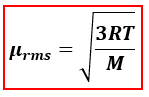# Problem: Calculate the root-mean-square speed of methane, CH4 (g), at 78 °C. (a) 23 m/s (b) 350 m/s(c) 550 m/s (d) 667 m/s (e) 739 m/s

###### FREE Expert Solution

We’re being asked to calculate for the root mean square speed of CH4 at 78°C.

When calculating for root mean square speed (μrms), we will use the following equation:µrms = rms speed,
R = 8.314 J/(mol·K)
T = temperature, K
M = molar mass, kg/mol

Given:###### Problem Details

Calculate the root-mean-square speed of methane, CH4 (g), at 78 °C.

(a) 23 m/s

(b) 350 m/s

(c) 550 m/s

(d) 667 m/s

(e) 739 m/s

What scientific concept do you need to know in order to solve this problem?

Our tutors have indicated that to solve this problem you will need to apply the Root Mean Square Speed concept. You can view video lessons to learn Root Mean Square Speed. Or if you need more Root Mean Square Speed practice, you can also practice Root Mean Square Speed practice problems.

What is the difficulty of this problem?

Our tutors rated the difficulty ofCalculate the root-mean-square speed of methane, CH4 (g), at...as medium difficulty.

How long does this problem take to solve?

Our expert Chemistry tutor, Dasha took 5 minutes and 5 seconds to solve this problem. You can follow their steps in the video explanation above.

What professor is this problem relevant for?

Based on our data, we think this problem is relevant for Professor Davis' class at UCF.